This tool helps you understand and debug math code written in the syntax of popular computer programming languages like MATLAB, C, Java, Python, and R. It comes in handy when you're reading other people's code, especially when it contains nested parentheses, fractions, and exponents.

Paste a line of code that represents a math expression:

Example equations:

These equations were taken directly from real MATLAB code. As you can see, they are much easier to understand and debug when visualized using MathViz.

• 1/(4*pi*(2*pi*f))*pr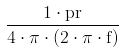• 2*a/b*c*(sin(pi*c/2)/(pi*c)/2)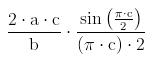• (-1./(sqrt(-1)*2*pi*freq(k)*rho)).*(p1(:,k) - p2(:,k));• 3/(2*pi*(numel(krs)-1)*log10(exp(1)))*(krs(end) - krs(1))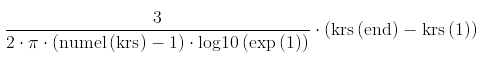• 1/2*(real(pressure_recreated1{i_freq}.*conj(vr_recreated{i_freq})))• max(ceil( (2*pi*oasp_input.f/oasp_input.wi_cmin - 2*pi*oasp_input.f/oasp_input.wi_cmax)/ (pi/4/(Rmax*1000)) ), 10);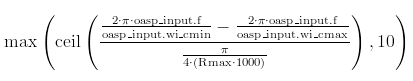This pair shows how one tiny change in MATLAB code can totally alter the expression's meaning, something that is very easy to see in MathViz:
• finite_diff = (func(x+delta)-func(x-delta))/(delta*2)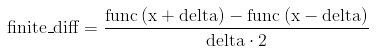• finite_diff = (func(x+delta)-func(x-delta))/delta*2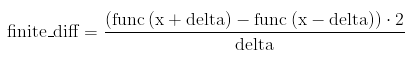Here's a nasty-looking MATLAB expression that MathViz helped Kevin to understand. He told me over email: "Like, there's no way you could parse that in your head without spending a good 30 seconds looking at it even though it's a small equation. But mathviz makes it easy to understand in quicker than you can say 'latex'!"
• ((ws./c).*(1-1/2*(2./((ws./c)*d)).^2))Currently supports:
• MATLAB and C-style variable names: my_var_1
• struct variables: record.field.sub_field
• R data frame variables: frame\$field
• flattening of fractions: x / y / z / w
• exponents: a^b
• square root: sqrt(x/y)
• unary minus: -x
• parentheses: 2*(3*4)
• comparisons: x <= 3 / y
• array accesses using parens and braces (MATLAB) and brackets (C-style): A(5), A{5}, A
• function calls: fib(n-1) + fib(n-2)
• elimination of redundant parentheses: ((x + y)) ^ (((z + w)))
• MATLAB array accesses using colons and commas: A(i,j,k,:)
• Python array accesses and slicing: A[3:-2]
• MATLAB left divide: y \ x
• MATLAB dot operators: x.*y, A.^b
• MATLAB transpose: A.'
• MATLAB Hermitian transpose: A' (same as A.' for real matrices)
Possible new features (if there is a demand):
• One-click feedback mechanism for queries that are rendered incorrectly
• Multi-dimensional arrays: A
• Selective rendering of an entire source file, not just of one line of code

MathViz was created by Philip Guo. If you have questions, bug reports, or suggestions, please contact me at philip@pgbovine.net

Special thanks to Kevin Cockrell for creating an early prototype, inspiring the creation of MathViz, doing beta testing, making feature requests, and providing example MATLAB code.